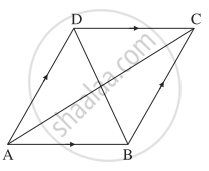# In a parallelogram ABCD, diagonal vectors are bar"AC" = 2hat"i" + 3hat"j" + 4hat"k" and bar"BD" = - 6hat"i" + 7hat"j" - 2hat"k", then find the adjacent side vectors bar"AB" and bar"AD". - Mathematics and Statistics

Sum

In a parallelogram ABCD, diagonal vectors are bar"AC" = 2hat"i" + 3hat"j" + 4hat"k" and bar"BD" = - 6hat"i" + 7hat"j" - 2hat"k", then find the adjacent side vectors bar"AB" and bar"AD".

#### SolutionABCD is a parallelogram.

∴ bar"AB" = bar"DC", bar"AD" = bar"BC"

bar"AC" = bar"AB" + bar"BC"

= bar"AB" + bar"AD"     ...(1)

bar"BD" = bar"BA" + bar"AD" = - bar"AB" + bar"AD"    ...(2)

Adding (1) and (2), we get

2bar"AD" = bar"AC" + bar"BD" = (2hat"i" + 3hat"j" + 4hat"k") + (- 6hat"i" + 7hat"j" - 2hat"k")

= - 4hat"i" + 10hat"j" + 2hat"k"

∴ bar"AD" = 1/2(- 4hat"i" + 10hat"j" + 2hat"k")

= - 2hat"i" + 5hat"j" + hat"k"

From (1), bar"AB" = bar"AC" - bar"AD"

= (2hat"i" + 3hat"j" + 4hat"k") - (- 2hat"i" + 5hat"j" + hat"k")

= 4hat"i" - 2hat"j" + 3hat"k"

#### Notes

[Note: Answer in the textbook is incorrect.]

Concept: Vectors and Their Types
Is there an error in this question or solution?